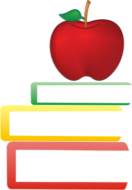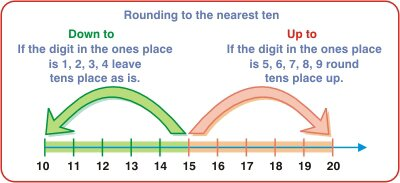2nd Grade Math Learning - Math Worksheets and Activities - MyHomeSchoolMath

myhomeschoolmath.comTitle
Go to content2nd Grade Math Learning - Math Worksheets and Activities

Free printable Math Worksheets - 2nd grade

This is a collection of math worksheets for grade 2, organized by topics such as comparing, rounding, place value, addition, subtraction, adding and subtracting in columns, mental math, multiplication, division, measuring, and geometry.
The worksheets are, except in pdf form, randomly generated each time you click on the links below. You can also get a new, different one just by refreshing the page in your browser.
Numbers up to 100, Rounding to the nearest tenSubtraction